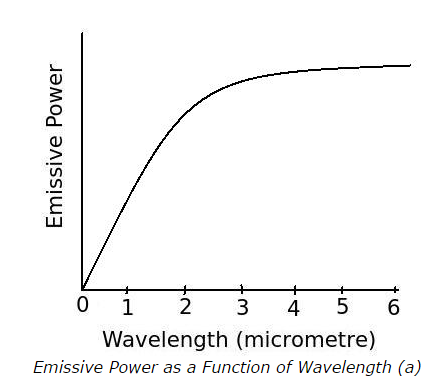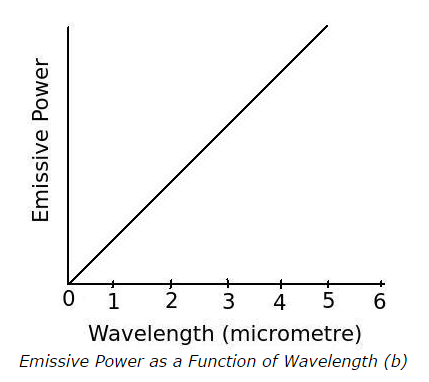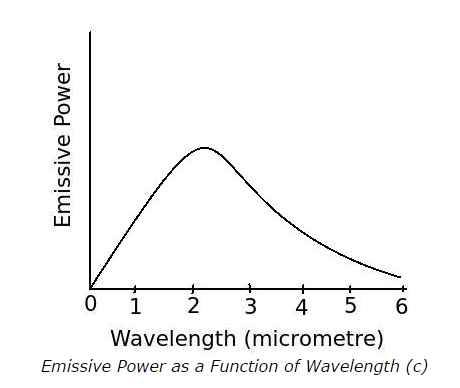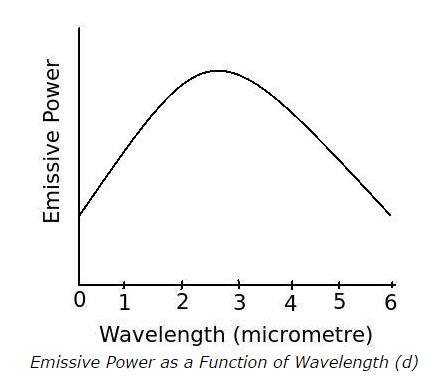1. What is emissive power of a body?

a. total radiation emitted by the body per unit volume and time
b. total radiation emitted by the body per unit temperature and time
c. total radiation emitted by the body per unit area and time
d. none of the above

ANSWER: c. total radiation emitted by the body per unit area and time

2. The emissive power of a body depends upon

a. temperature of the the body
b. characteristics of the surface of the body
c. both a. and b.
d. none of the above

ANSWER: c. both a. and b.

3. At a given temperature if wavelengths are changed, the quality of radiation per unit wavelength

a. will always be constant
b. will also be changed
c. will be changed sometimes but sometimes be constant
d. unpredictable

ANSWER: b. will also be changed

4. How is the relation between emissive power of a body and the radiation wavelength represented for an ideal radiator at a given temperature say 1900 K?

a.b.c.d.5. What is the relation between temperature of a body and the wavelength of its emitted radiation at its maximum emissive power?

a. as temperature of a body increases the wavelength of its emitted radiation also increases at its maximum emissive power
b. as temperature of a body increases the wavelength of its emitted radiation decreases at its maximum emissive power
c. as temperature of a body increases the wavelength of its emitted radiation does not change only at its maximum emissive power
d. none of the above

ANSWER: b. as temperature of a body increases the wavelength of its emitted radiation decreases at its maximum emissive power

6. Which of the following relation is correct for the radiation?

7. What is the relation between reflectivity (ρ), absorptivity (α) and transmissivity (τ)?

a. ρ – α + τ = 1
b. ρ + α – τ = 1
c. ρ + α + τ = 1
d. ρ – α – τ = 1

ANSWER: c. ρ + α + τ = 1

8. What is the opaque body?

a. the body which does not reflect radiation
b. the body which does not absorb radiation
c. the body which does not transmit radiation
d. all of the above

9. What is diffuse reflection of radiation?

a. the reflection of radiation on a rough surface which occurs in all directions
b. the reflection of radiation on a rough surface which occurs in a single direction
c. the reflection of radiation on a smooth surface
d. none of the above

ANSWER: a. the reflection of radiation on a rough surface which occurs in all directions

10. The reflection in which the angle of incident is equal to the angle of reflection is called as

a. regular reflection
b. specular reflection
c. both a. and b.
d. none of the above

ANSWER: c. both a. and b.

11. The values of reflectivity (ρ), absorptivity (α) and transmissivity (τ) of a body are depend upon

a. material of the body
b. surface condition of the body